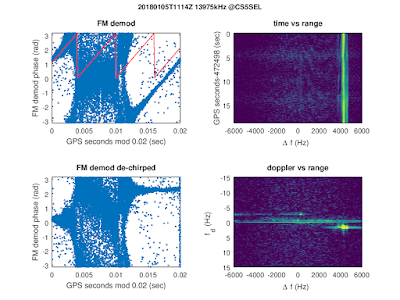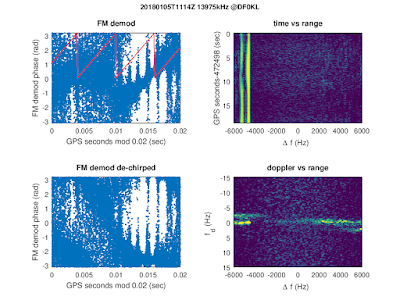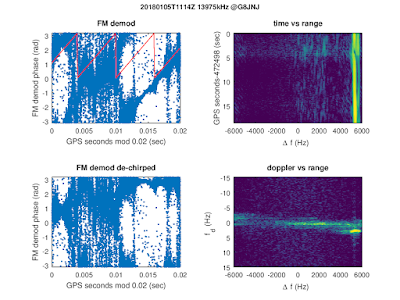## Wednesday, January 24, 2018

### Using an extended Kalman filter for TDoA HF geo-location

In this post the precision of geo-location using TDoA on shortwave is explored using the data shown on this blog before.

The idea is to feed the time series of time differences into an extended Kalman filter (EKF) using two models for the propagation time from the transmitter to the receiver:

1) Simple ray-geometry for oblique propagation in the ionosphere:
• based on arXiv:1104.2248 which uses the Breit and Tuve + Martyn theorems to convert vertical to oblique incidence
• using vertical electron density profiles from the IRI2016 model as an input
• group refractive index: 1/sqrt(1-fN2/f2), i.e., no magentoionic effects are taken into account
• when integrating over the group refractive index in the vertical direction, analytic integration of 1/sqrt(f(h)) is performed in each height interval in order to avoid the spurious numerical singularity at the reflection point
• spherical coordinates are used (rE=6371km)
2) Alternatively, great-circle distances on the WGS84 ellipsoid are used:

All receiver coordinates are assumed to be in WGS84, however in practice it is suspected that there are deviations from the true locations of up to 20 km, as the KiwiSDR positions are provided by the KiwiSDR owners and are not taken from the GPS position solutions used for time synchronization.

The plot below shows the output of the EKF
• implementation in octave following ADA285972
• state = [lat, lon] of the unknown position
• measurements = time differences between pairs of KiwiSDRs
• the measurements covariance matrix (constant) is build from the covariances of time differences (and is set to zero for time differences not sharing a common receiver)
• state covariance matrix = diag(0.01, 0.01)2 deg2 (constant)
• start covariance = diag(0.1, 0.1)2 deg2
• there are about 60 measurements which are run 5 times sequentially through the EKFExtended Kalman Filter (EKF) output; note that the range of the x(y) axis corresponds to approximately 17(67)km.

The simple ionosphere propagation model improves the match to the known position but there is still a remaining bias of about 30km North, 4km West. Clearly, more studies are needed in order to find out if this improvement is by chance or real.

## Friday, January 5, 2018

### De-chirping applied to a HF over the horizon radar signal (2)

This is a follow up to this blog post. Again the signals of the OTHR in Cyprus were analyzed using GPS time-stamped IQ samples from several KiwiSDRs.

In this case the OTHR signal was found between 13965 and 13985 kHz. The slope of the FMCW signal is μ=1MHz/s, and there are 50 sweeps per second. This results in a bandwidth of about 20kHz.

As is well known, the frequencies Δf of the spectrum of the de-chirped signal correspond to time delays τ=-Δf/μ in the case of a stationary target.
• for this one has to multiply the received signal with the original waveform
• tuning offsets w.r.t. center frequency of the OTHR signal correspond to a time delay of the original waveform
• when Δf is positive the delay corresponds to the frequency Δf-fs (fs is the sampling frequency), i.e. the ranges wrap around the x-axis.
Applying a 2nd Fourier transform to the time direction of time vs. Δf data one obtains the Doppler frequency fd vs. Δf where fd = v•λ/2 (for velocity v and wavelength λ). Here one has to be careful to adjust the phases in each bin to correct for the fact that the sampling times are not exactly aligned to multiples of 0.02 seconds.

Plots for 5 KiwiSDR are shown below. The splitting of the ionospheric reflection is most likely due to O- and X-mode propagation in the F layer.13975 kHz 20180105T1114Z @CS5SEL; the band at fd=0 is caused by an interference13975 kHz 20180105T1114Z @DF0KL13975 kHz 20180105T1114Z @G8JNJ13975 kHz 20180105T1114Z @Izhvesk13975 kHz 20180105T1114Z @Julisdalen
Thanks to all KiwiSDR owners who make their receivers available and do connect a GPS antenna.